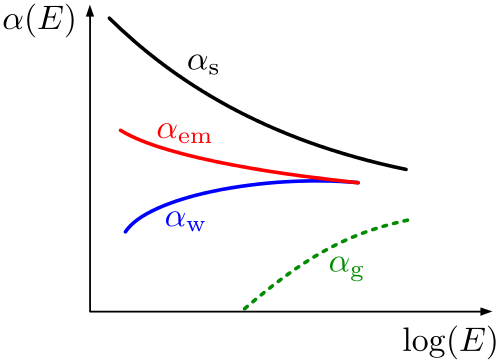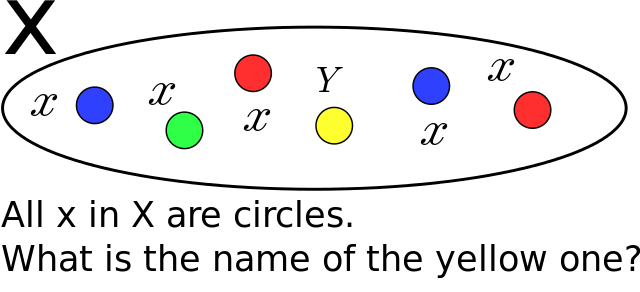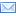Difference Between Similar Terms and Objects

Difference Between Constants and VariablesWhat is Constants?

Constants are a type of value used most often in mathematical expressions and equations. Like the name implies, constants do not change in value over time. Constants can be any type of number (not just an integer or fraction, for instance).What is Variables?

Variables are symbols that act as placeholders for numbers (or, potentially, strings and other data). Variables are usually visualized as letters or a phrase, and in certain cases variables can have more than one possible value. Most often variables are used to indicate that a number in an equation or expression is not yet known.

Difference between Constants and Variables

Symbolization of  Constants and Variables

1. Constants are usually written as a number, such as 12 or -4.23. However, some important constants can have names and unique symbols that are recognizable throughout mathematics and sciences. For instance, pi (symbolized as π) is a common constant in geometry, calculus, and other sciences. Because pi is an irrational number with a decimal expansion that continues infinitely without a repeating pattern, it cannot be fully written and is therefore simply indicated by its symbol or name.

2. Theoretically, a variable can be symbolized by anything except a constant number. Variables are most commonly written as a single letter, especially x and y. These letters can be chosen at random, and are simply placeholders for the actual value that the variable represents. In computer programming, variables are more commonly written as a phrase that refers to the purpose of the variable (such as bonusDollars or numberOfEmployees).

Characteristics of Constants and Variables in Mathematical

1. In a mathematical expression, a constant is a number that does not change in value. For example, in the equation x + 5 = 7, 5 and 7 are both constants (while x is a variable).

2. A variable in an equation is a number that has not yet been determined. As an example, consider the following:

y + 4 = 9

In this equation, y is an unknown value. Solving the equation returns a value of 5 for y. Multiple variables can be used in the same equation, which usually increases the number of the variables’ possible values. Consider the next equation:

y + 4 = z

This equation returns an infinite number of possible values for both y and z (5 and 9, 6 and 10, -1 and 3, etc.) Because of these infinite possibilities, equations with multiple variables are typically presented in a system of equations, or a set of multiple equations, to determine a minimum number of useful values. Variables can also be used in more specific types of equations, such as in statistical regression models, where the beta coefficients act as constants and the beta variables can be changed to determine the dependent variable given a certain set of real world conditions. A beta variable could be price, gross domestic product, inflation rate, or any other condition whose changes over time affect the dependent variable in question.

Characteristics of Constants and Variables in Computer Programming

1. The use of constants in programming is identical to their use in mathematics. They are typically displayed as numbers and can be used in equations and expressions. For example, take the following line of code:

totalStaff = 5 + numberOfInterns

In this equation, totalStaff and numberOfInterns are both variables, but 5 is an unchanging constant. (For the purpose of this code, the constant 5 could refer to the number of permanent staff positions in a company – a number that would not change randomly.)

A constant can be defined in some programming languages. This is very similar to defining a variable, in which the constant is given a symbol or name that can be referred to over and over in the code. However, a constant cannot be redefined in a later piece of code; it must retain its original value. This allows the code writer to signify that the same constant (such as pi or a sales tax rate) is being used again, but the code compiler will not look for changes in the value of the constant, which can save on computation time.

A constant in computer programming does not have to be defined as numerical data, but can be any other data that is unchanging, like a string of words or letters.

2. Variables in computer programming have more uses and manifestations than in traditional mathematics and sciences. In programming, a variable can be a placeholder for any information, not just numbers. Variables can also be used to indicate strings, arrays, and other types of data. Take the following code:

define variable ExampleVar
ExampleVar = 3

In this example (generic code not specific to any programming language), the first line tells the program compiler that we are defining (or creating) a variable called ExampleVar. In the second line, we set the variable equal to the integer 3. We could also write this variation:

ExampleVar = “Hello world”

This is still a variable, but unlike in a mathematical equation, the variable is equal to a string of letters. Because we have defined ExampleVar as a variable and not a constant, at any point in the code ExampleVar could be redefined (although in most programming languages, the new value of the variable must stay the same type of information, like a new integer or string).

Summary of  Constants and Variables

Constants and variables can both be loosely defined as elements of equations and expressions that represent certain values. However, they are distinct, complementary concepts that have important differences in their uses.

• Constants do not change over time. They are by definition numbers (or data) that are unchanging in an equation, expression, or piece of programming. Variables represent unknown or changing values.
• In math, constants are simply written as numbers, while variables are signified by letters or symbols. Variables typically represent unknown values or values that can be changed to reflect scientific conditions.
• Constants and variables have extra uses in computer programming compared to their uses in mathematics and sciences. Variables can be numbers, strings, or other information. A constant can be given a name or symbol just like a variable in most languages, but the value of the constant cannot be changed in later lines of code.

Comparison table for Constants and VariablesSearch DifferenceBetween.net :

Custom Search

Help us improve. Rate this post!Loading...Email This Post : If you like this article or our site. Please spread the word. Share it with your friends/family.

1 Comment

1. Extremely good

Please note: comment moderation is enabled and may delay your comment. There is no need to resubmit your comment.

References :

Jari Koopman. “What is the difference between constant and variable.” Treehouse. December 9, 2015. https://teamtreehouse.com/community/what-is-difference-between-constant-and-variable

Jesse Liberty and Rogers Cadenhead. “Creating Variables and Constants in C++.” InformIT. May 3, 2011. http://www.informit.com/articles/article.aspx?p=1703469&seqNum=5

Uri (answerer username). “Why use constants in programming?” StackOverflow. June 1, 2010. https://stackoverflow.com/questions/2953601/why-use-constants-in-programming

“C Programming Constants and Variables.” Learn C. https://www.programiz.com/c-programming/c-variables-constants

“C Tutorial – variables and constants.” CodingUnit Programming Tutorials. https://www.codingunit.com/c-tutorial-variables-and-constants

Articles on DifferenceBetween.net are general information, and are not intended to substitute for professional advice. The information is "AS IS", "WITH ALL FAULTS". User assumes all risk of use, damage, or injury. You agree that we have no liability for any damages.